# [PoreSpy] 图像导入和基本处理

## 1 图像导入

### 1.1 2D图像

CT扫描的图像是连续的二维切片，将这些图片放进一个文件夹里，通过特定的Python函数可以导入，

import imageio.v2 as imageio
import porespy as ps
import numpy as np
from matplotlib.pyplot import subplots

seq = io.imread_collection("C:/Users/ALPHA/Desktop/PNM\object/test3-2d/*.tif") #读取文件夹里的tif文件并以列表的方式存储
seq.files

'''
['C:/Users/ALPHA/Desktop/PNM/object/test3-2d\\test3-2D000.tif',
'C:/Users/ALPHA/Desktop/PNM/object/test3-2d\\test3-2D001.tif',
'C:/Users/ALPHA/Desktop/PNM/object/test3-2d\\test3-2D002.tif',
.....
'C:/Users/ALPHA/Desktop/PNM/object/test3-2d\\test3-2D297.tif',
'C:/Users/ALPHA/Desktop/PNM/object/test3-2d\\test3-2D298.tif',
'C:/Users/ALPHA/Desktop/PNM/object/test3-2d\\test3-2D299.tif']
'''

def imread_collection(load_pattern, conserve_memory=True,
plugin=None, **plugin_args):
"""

Parameters
----------
List of objects to load. These are usually filenames, but may
vary depending on the currently active plugin.  See the docstring
for ImageCollection for the default behaviour of this parameter.
conserve_memory : bool, optional
If True, never keep more than one in memory at a specific
time.  Otherwise, images will be cached once they are loaded.

Returns
-------
ic : ImageCollection
Collection of images.

Other Parameters
----------------
plugin_args : keywords
Passed to the given plugin.

"""


fig, ax = subplots(figsize=[5, 5])
im1=seq
ax.imshow(im1,cmap='gray');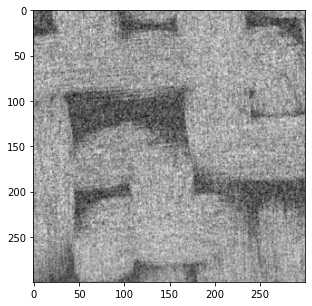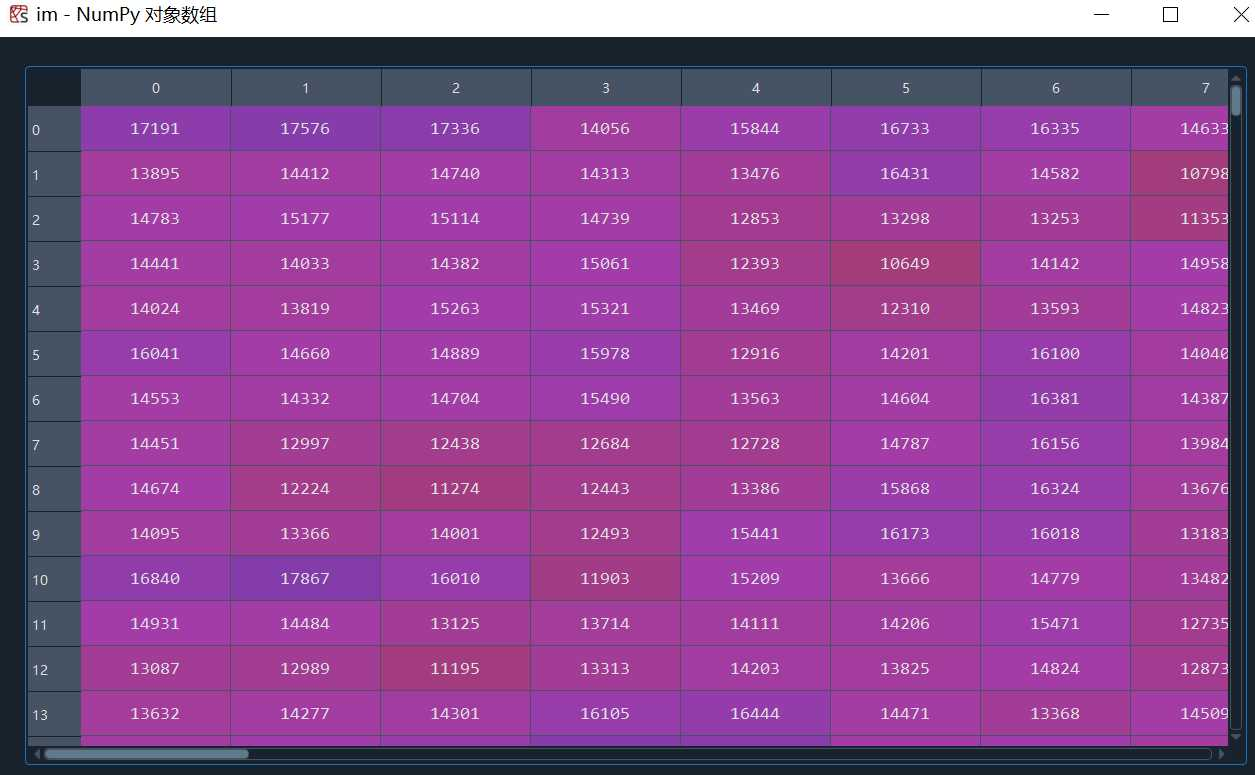### 1.2 2D文件夹转3D文件

#遍历文件夹中的所有切片，取每个切片的xz，yz面，合并成整个模型的xz，yz面，得到模型的三维图片
im3d = np.zeros([*seq.shape, len(seq)])
for i, im in enumerate(seq):
im3d[..., i] = im
fig, ax = subplots(figsize=[5, 5])
ax.imshow(ps.visualization.show_planes(im3d),cmap='gray');imageio.volsave('C:/Users/ALPHA/Desktop/PNM/object/im3d.tif',np.array(im3d, dtype=np.int8))
#volsave = volwrite
def volwrite(uri, im, format=None, **kwargs):
"""volwrite(uri, vol, format=None, **kwargs)

Write a volume to the specified file.

Parameters
----------
uri : {str, pathlib.Path, file}
The resource to write the image to, e.g. a filename, pathlib.Path
vol : numpy.ndarray
The image data. Must be NxMxL (or NxMxLxK if each voxel is a tuple).
format : str
The format to use to read the file. By default imageio selects
the appropriate for you based on the filename and its contents.
kwargs : ...
Further keyword arguments are passed to the writer. See :func:.help
to see what arguments are available for a particular format.
"""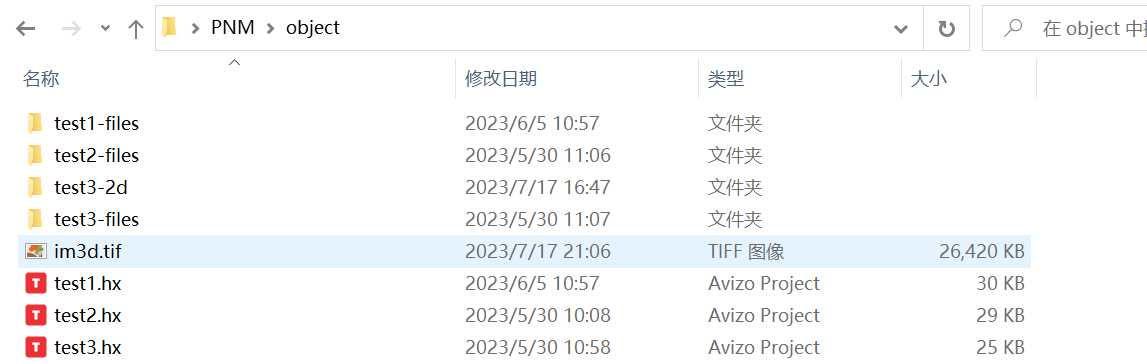### 1.2 直接导入3D图像

im2 = imageio.volread('C:/Users/ALPHA/Desktop/PNM/object/test3-3D.tif')
ps.imshow(im2, axis=0,cmap='gray');

Reads a volume from the specified file. Returns a numpy array, which
comes with a dict of meta data at its 'meta' attribute.

Parameters
----------
uri : {str, pathlib.Path, bytes, file}
The resource to load the volume from, e.g. a filename, pathlib.Path,
format : str
The format to use to read the file. By default imageio selects
the appropriate for you based on the filename and its contents.
kwargs : ...
Further keyword arguments are passed to the reader. See :func:.help
to see what arguments are available for a particular format.
"""## 2 图像处理

CT产生的图像都是灰度值图象的，每个像素都包含一个数值，指示该位置的强度。在理想的图片中，灰度值将很好地分布，使得对应于固体框架的所有明亮像素都具有高灰度值（即200-255），而对应于孔隙的所有暗像素都具有低灰度值值（即0-50）。如果是这种情况，区分或分割固体和孔隙隙将非常容易。在PoreSpy和imageio里图像为灰度值图像，以储存

### 2.1 图像的噪声

import imageio.v2 as imageio
import porespy as ps
import numpy as np
from matplotlib.pyplot import subplots

im = ps.generators.blobs(shape=[100, 100])

new_im = (im*0.2) * np.random.rand(*im.shape) + 0.8*im
new_im += (~im*0.2) * np.random.rand(*im.shape)
fig, ax = subplots(figsize=[5, 5])
ax.imshow(new_im,cmap='gray');

#ps.generators.blobs()
def blobs(
shape: List[int],
porosity: float = 0.5,
blobiness: int = 1,
divs: int = 1,
seed=None,
):
"""
Generates an image containing amorphous blobs

Parameters
----------
shape : list
The size of the image to generate in [Nx, Ny, Nz] where N is the
number of voxels
porosity : float
If specified, this will threshold the image to the specified value
prior to returning.  If None is specified, then the scalar
noise field is converted to a uniform distribution and returned
without thresholding.
blobiness : int or list of ints(default = 1)
Controls the morphology of the blobs.  A higher number results in
a larger number of small blobs.  If a list is supplied then the
blobs are anisotropic.
divs : int or array_like
The number of times to divide the image for parallel processing.
If 1 then parallel processing does not occur.  2 is
equivalent to [2, 2, 2] for a 3D image.  The number of cores
used is specified in porespy.settings.ncores and defaults to
all cores.
seed : int, optional, default = None
Initializes numpy's random number generator to the specified state. If not
provided, the current global value is used. This means calls to
np.random.state(seed) prior to calling this function will be respected.

Returns
-------
image : ndarray
A boolean array with True values denoting the pore space

--------
norm_to_uniform

Notes
-----
This function generates random noise, the applies a gaussian blur to
the noise with a sigma controlled by the blobiness argument as:

np.mean(shape) / (40 * blobiness)

The value of 40 was chosen so that a blobiness of 1 gave a
reasonable result.

Examples
--------
Click here
_
to view online example.

"""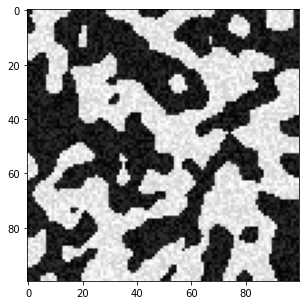fig, ax = subplots(figsize=[5, 5])
ax.hist(new_im.flatten(), bins=25, edgecolor='k');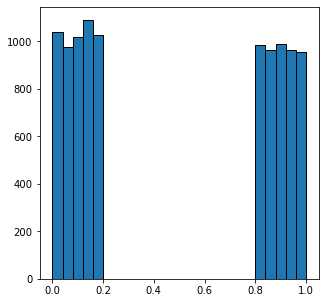fig, ax = subplots(figsize=[5, 5])
ax.imshow(new_im > 0.5,cmap='gray');new_im = np.random.normal(loc=.4, scale=0.1, size=im.shape) * im
new_im += np.random.normal(loc=.6, scale=0.1, size=im.shape) * (~im)
fig, ax = subplots(1, 3, figsize=[15, 5])
ax.imshow(new_im)
ax.hist(new_im.flatten(), bins=25, edgecolor='k')
ax.imshow(new_im > 0.5);### 2.2 噪声过滤

#### 2.2.1 不保留边缘的过滤方式

import scipy.ndimage as spim
im = (im - im.min())/(im.max() - im.min())

im1 = spim.uniform_filter(im, size=3)
im1 = (im1 - im1.min())/(im1.max() - im1.min())
im2 = spim.gaussian_filter(im, sigma=1)
im2 = (im2 - im2.min())/(im2.max() - im2.min())
fig, ax = subplots(2, 3, figsize=[10, 5])
ax.imshow(im,cmap='gray')
ax.imshow(im1,cmap='gray')
ax.imshow(im2,cmap='gray')
ax.imshow(im > 0.6,cmap='gray')
ax.imshow(im1 > 0.6,cmap='gray')
ax.imshow(im2 > 0.6,cmap='gray');

#spim.uniform_filter()
def uniform_filter(input, size=3, output=None, mode="reflect",
cval=0.0, origin=0, *, axes=None):
"""Multidimensional uniform filter.

Parameters
----------
%(input)s
size : int or sequence of ints, optional
The sizes of the uniform filter are given for each axis as a
sequence, or as a single number, in which case the size is
equal for all axes.
%(output)s
%(mode_multiple)s
%(cval)s
%(origin_multiple)s
axes : tuple of int or None, optional
If None, input is filtered along all axes. Otherwise,
input is filtered along the specified axes. When axes is
specified, any tuples used for size, origin, and/or mode
must match the length of axes. The ith entry in any of these tuples
corresponds to the ith entry in axes.

Returns
-------
uniform_filter : ndarray
Filtered array. Has the same shape as input.

Notes
-----
The multidimensional filter is implemented as a sequence of
1-D uniform filters. The intermediate arrays are stored
in the same data type as the output. Therefore, for output types
with a limited precision, the results may be imprecise because
intermediate results may be stored with insufficient precision.

Examples
--------
>>> from scipy import ndimage, datasets
>>> import matplotlib.pyplot as plt
>>> fig = plt.figure()
>>> plt.gray()  # show the filtered result in grayscale
>>> ax1 = fig.add_subplot(121)  # left side
>>> ax2 = fig.add_subplot(122)  # right side
>>> ascent = datasets.ascent()
>>> result = ndimage.uniform_filter(ascent, size=20)
>>> ax1.imshow(ascent)
>>> ax2.imshow(result)
>>> plt.show()
"""


#spim.gaussian_filter()
def gaussian_filter(input, sigma, order=0, output=None,
axes=None):
"""Multidimensional Gaussian filter.

Parameters
----------
%(input)s
sigma : scalar or sequence of scalars
Standard deviation for Gaussian kernel. The standard
deviations of the Gaussian filter are given for each axis as a
sequence, or as a single number, in which case it is equal for
all axes.
order : int or sequence of ints, optional
The order of the filter along each axis is given as a sequence
of integers, or as a single number. An order of 0 corresponds
to convolution with a Gaussian kernel. A positive order
corresponds to convolution with that derivative of a Gaussian.
%(output)s
%(mode_multiple)s
%(cval)s
truncate : float, optional
Truncate the filter at this many standard deviations.
Default is 4.0.
radius : None or int or sequence of ints, optional
Radius of the Gaussian kernel. The radius are given for each axis
as a sequence, or as a single number, in which case it is equal
for all axes. If specified, the size of the kernel along each axis
will be 2*radius + 1, and truncate is ignored.
Default is None.
axes : tuple of int or None, optional
If None, input is filtered along all axes. Otherwise,
input is filtered along the specified axes. When axes is
specified, any tuples used for sigma, order, mode and/or radius
must match the length of axes. The ith entry in any of these tuples
corresponds to the ith entry in axes.

Returns
-------
gaussian_filter : ndarray
Returned array of same shape as input.

Notes
-----
The multidimensional filter is implemented as a sequence of
1-D convolution filters. The intermediate arrays are
stored in the same data type as the output. Therefore, for output
types with a limited precision, the results may be imprecise
because intermediate results may be stored with insufficient
precision.

The Gaussian kernel will have size 2*radius + 1 along each axis. If
radius is None, the default radius = round(truncate * sigma) will be
used.

Examples
--------
>>> from scipy.ndimage import gaussian_filter
>>> import numpy as np
>>> a = np.arange(50, step=2).reshape((5,5))
>>> a
array([[ 0,  2,  4,  6,  8],
[10, 12, 14, 16, 18],
[20, 22, 24, 26, 28],
[30, 32, 34, 36, 38],
[40, 42, 44, 46, 48]])
>>> gaussian_filter(a, sigma=1)
array([[ 4,  6,  8,  9, 11],
[10, 12, 14, 15, 17],
[20, 22, 24, 25, 27],
[29, 31, 33, 34, 36],
[35, 37, 39, 40, 42]])

>>> from scipy import datasets
>>> import matplotlib.pyplot as plt
>>> fig = plt.figure()
>>> plt.gray()  # show the filtered result in grayscale
>>> ax1 = fig.add_subplot(121)  # left side
>>> ax2 = fig.add_subplot(122)  # right side
>>> ascent = datasets.ascent()
>>> result = gaussian_filter(ascent, sigma=5)
>>> ax1.imshow(ascent)
>>> ax2.imshow(result)
>>> plt.show()
"""


gaussian 高斯滤波原理可参考：高斯滤波gaussian_filter()_gaussian filter_wanghua609的博客-CSDN博客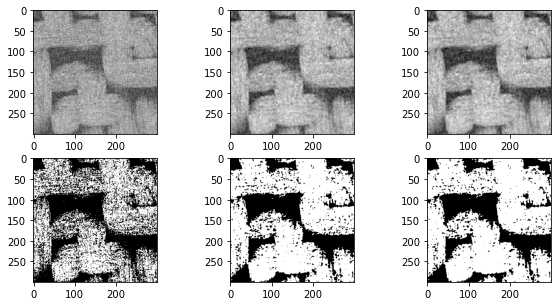im = ~ps.generators.random_spheres([100, 100], r=8)*1.0
im1 = spim.uniform_filter(im, size=6)
im2 = spim.gaussian_filter(im, sigma=2)
fig, ax = subplots(2, 2, figsize=[10, 10])
ax.imshow(im1,cmap='gray')
ax.imshow(im2,cmap='gray')
ax.imshow(im1 > im1.mean(),cmap='gray')
ax.imshow(im2 > im2.mean(),cmap='gray');#### 2.2.2 保留边缘的过滤方式

import imageio.v2 as imageio
import porespy as ps
import numpy as np
from matplotlib.pyplot import subplots
import scipy.ndimage as spim
from skimage.restoration import non_local_means, estimate_sigma

im = (im - im.min())/(im.max() - im.min())

im1 = spim.median_filter(im, size=3)
im1 = (im1 - im1.min())/(im1.max() - im1.min())
s = estimate_sigma(im)
im2 = non_local_means.denoise_nl_means(im)
im2 = (im2 - im2.min())/(im2.max() - im2.min())
fig, ax = subplots(2, 3, figsize=[10, 5])
ax.imshow(im,cmap='gray')
ax.imshow(im1,cmap='gray')
ax.imshow(im2,cmap='gray')
ax.imshow(im > 0.6,cmap='gray')
ax.imshow(im1 > 0.6,cmap='gray')
ax.imshow(im2 > 0.5,cmap='gray');

def median_filter(input, size=None, footprint=None, output=None,
mode="reflect", cval=0.0, origin=0, *, axes=None):
"""
Calculate a multidimensional median filter.

Parameters
----------
%(input)s
%(size_foot)s
%(output)s
%(mode_reflect)s
%(cval)s
%(origin_multiple)s
axes : tuple of int or None, optional
If None, input is filtered along all axes. Otherwise,
input is filtered along the specified axes.

Returns
-------
median_filter : ndarray
Filtered array. Has the same shape as input.

--------
scipy.signal.medfilt2d

Notes
-----
For 2-dimensional images with uint8, float32 or float64 dtypes
the specialised function scipy.signal.medfilt2d may be faster. It is
however limited to constant mode with cval=0.

Examples
--------
>>> from scipy import ndimage, datasets
>>> import matplotlib.pyplot as plt
>>> fig = plt.figure()
>>> plt.gray()  # show the filtered result in grayscale
>>> ax1 = fig.add_subplot(121)  # left side
>>> ax2 = fig.add_subplot(122)  # right side
>>> ascent = datasets.ascent()
>>> result = ndimage.median_filter(ascent, size=20)
>>> ax1.imshow(ascent)
>>> ax2.imshow(result)
>>> plt.show()
"""fig, ax = subplots(1, 3, figsize=[15, 5])
ax.hist(im.flatten(), bins=25, edgecolor='k')
ax.hist(im1.flatten(), bins=25, edgecolor='k')
ax.hist(im2.flatten(), bins=25, edgecolor='k');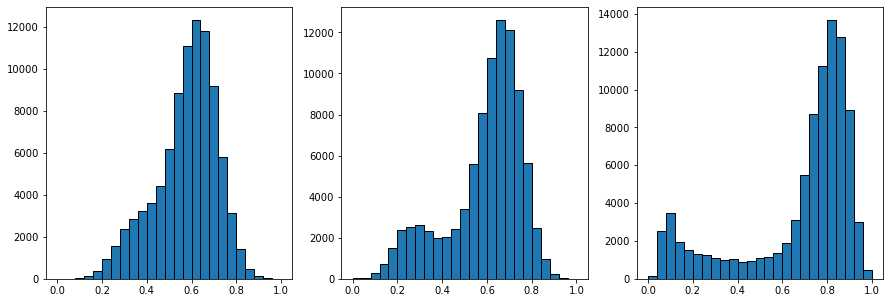im = imageio.volread('C:/Users/ALPHA/Desktop/PNM\object/test3-2d/test3-2D000.tif')
im = (im - im.min())/(im.max() - im.min())
s = estimate_sigma(im)

kw = {
'patch_size': 7,
'patch_distance': 11,
'h': 0.1,
}
im1 = non_local_means.denoise_nl_means(im, sigma=s, **kw)
im1 = (im1 - im1.min())/(im1.max() - im1.min())

kw = {
'patch_size': 7,
'patch_distance': 7,
'h': 0.1,
}
im2 = non_local_means.denoise_nl_means(im, sigma=s, **kw)
im2 = (im2 - im2.min())/(im2.max() - im2.min())

kw = {
'patch_size': 9,
'patch_distance': 7,
'h': 0.1,
}
im3 = non_local_means.denoise_nl_means(im, sigma=s, **kw)
im3 = (im3 - im3.min())/(im3.max() - im3.min())

fig, ax = subplots(2, 3, figsize=[10, 5])
ax.imshow(im,cmap='gray')
ax.imshow(im1,cmap='gray')
ax.imshow(im2,cmap='gray')
ax.imshow(im > 0.5,cmap='gray')
ax.imshow(im1 > 0.5,cmap='gray')
ax.imshow(im2 > 0.5,cmap='gray');

fig, ax = subplots(1, 3, figsize=[15, 5])
ax.hist(im.flatten(), bins=25, edgecolor='k')
ax.hist(im1.flatten(), bins=25, edgecolor='k')
ax.hist(im2.flatten(), bins=25, edgecolor='k');

#estimate_sigma
def estimate_sigma(image, average_sigmas=False, multichannel=False, *,
channel_axis=None):
"""
Robust wavelet-based estimator of the (Gaussian) noise standard deviation.

Parameters
----------
image : ndarray
Image for which to estimate the noise standard deviation.
average_sigmas : bool, optional
If true, average the channel estimates of sigma.  Otherwise return
a list of sigmas corresponding to each channel.
multichannel : bool
Estimate sigma separately for each channel. This argument is
deprecated: specify channel_axis instead.
channel_axis : int or None, optional
If None, the image is assumed to be a grayscale (single channel) image.
Otherwise, this parameter indicates which axis of the array corresponds
to channels.

channel_axis was added in 0.19.

Returns
-------
sigma : float or list
Estimated noise standard deviation(s).  If multichannel is True and
average_sigmas is False, a separate noise estimate for each channel
is returned.  Otherwise, the average of the individual channel
estimates is returned.

Notes
-----
This function assumes the noise follows a Gaussian distribution. The
estimation algorithm is based on the median absolute deviation of the
wavelet detail coefficients as described in section 4.2 of _.

References
----------
..  D. L. Donoho and I. M. Johnstone. "Ideal spatial adaptation
by wavelet shrinkage." Biometrika 81.3 (1994): 425-455.
:DOI:10.1093/biomet/81.3.425

Examples
--------
>>> import skimage.data
>>> from skimage import img_as_float
>>> img = img_as_float(skimage.data.camera())
>>> sigma = 0.1
>>> rng = np.random.default_rng()
>>> img = img + sigma * rng.standard_normal(img.shape)
>>> sigma_hat = estimate_sigma(img, channel_axis=None)

#non_local_means.denoise_nl_means()
def denoise_nl_means(image, patch_size=7, patch_distance=11, h=0.1,
multichannel=False, fast_mode=True, sigma=0., *,
preserve_range=False, channel_axis=None):
"""Perform non-local means denoising on 2D-4D grayscale or RGB images.

Parameters
----------
image : 2D or 3D ndarray
Input image to be denoised, which can be 2D or 3D, and grayscale
or RGB (for 2D images only, see multichannel parameter). There can
be any number of channels (does not strictly have to be RGB).
patch_size : int, optional
Size of patches used for denoising.
patch_distance : int, optional
Maximal distance in pixels where to search patches used for denoising.
h : float, optional
Cut-off distance (in gray levels). The higher h, the more permissive
one is in accepting patches. A higher h results in a smoother image,
at the expense of blurring features. For a Gaussian noise of standard
deviation sigma, a rule of thumb is to choose the value of h to be
sigma of slightly less.
multichannel : bool, optional
Whether the last axis of the image is to be interpreted as multiple
channels or another spatial dimension. This argument is deprecated:
specify channel_axis instead.
fast_mode : bool, optional
If True (default value), a fast version of the non-local means
algorithm is used. If False, the original version of non-local means is
used. See the Notes section for more details about the algorithms.
sigma : float, optional
The standard deviation of the (Gaussian) noise.  If provided, a more
robust computation of patch weights is computed that takes the expected
noise variance into account (see Notes below).
preserve_range : bool, optional
Whether to keep the original range of values. Otherwise, the input
image is converted according to the conventions of img_as_float.
Also see https://scikit-image.org/docs/dev/user_guide/data_types.html
channel_axis : int or None, optional
If None, the image is assumed to be a grayscale (single channel) image.
Otherwise, this parameter indicates which axis of the array corresponds
to channels.

channel_axis was added in 0.19.

Returns
-------
result : ndarray
Denoised image, of same shape as image.

Notes
-----

The non-local means algorithm is well suited for denoising images with
specific textures. The principle of the algorithm is to average the value
of a given pixel with values of other pixels in a limited neighbourhood,
provided that the *patches* centered on the other pixels are similar enough
to the patch centered on the pixel of interest.

In the original version of the algorithm _, corresponding to
fast=False, the computational complexity is::

image.size * patch_size ** image.ndim * patch_distance ** image.ndim

Hence, changing the size of patches or their maximal distance has a
strong effect on computing times, especially for 3-D images.

However, the default behavior corresponds to fast_mode=True, for which
another version of non-local means _ is used, corresponding to a
complexity of::

image.size * patch_distance ** image.ndim

The computing time depends only weakly on the patch size, thanks to
the computation of the integral of patches distances for a given
shift, that reduces the number of operations _. Therefore, this
algorithm executes faster than the classic algorithm
(fast_mode=False), at the expense of using twice as much memory.
This implementation has been proven to be more efficient compared to
other alternatives, see e.g. _.

Compared to the classic algorithm, all pixels of a patch contribute
to the distance to another patch with the same weight, no matter
their distance to the center of the patch. This coarser computation
of the distance can result in a slightly poorer denoising
performance. Moreover, for small images (images with a linear size
that is only a few times the patch size), the classic algorithm can
be faster due to boundary effects.

The image is padded using the reflect mode of skimage.util.pad
before denoising.

If the noise standard deviation, sigma, is provided a more robust
computation of patch weights is used.  Subtracting the known noise variance
from the computed patch distances improves the estimates of patch
similarity, giving a moderate improvement to denoising performance _.
It was also mentioned as an option for the fast variant of the algorithm in
_.

When sigma is provided, a smaller h should typically be used to
avoid oversmoothing.  The optimal value for h depends on the image
content and noise level, but a reasonable starting point is
h = 0.8 * sigma when fast_mode is True, or h = 0.6 * sigma when
fast_mode is False.

References
----------
..  A. Buades, B. Coll, & J-M. Morel. A non-local algorithm for image
denoising. In CVPR 2005, Vol. 2, pp. 60-65, IEEE.
:DOI:10.1109/CVPR.2005.38

..  J. Darbon, A. Cunha, T.F. Chan, S. Osher, and G.J. Jensen, Fast
nonlocal filtering applied to electron cryomicroscopy, in 5th IEEE
International Symposium on Biomedical Imaging: From Nano to Macro,
2008, pp. 1331-1334.
:DOI:10.1109/ISBI.2008.4541250

..  Jacques Froment. Parameter-Free Fast Pixelwise Non-Local Means
Denoising. Image Processing On Line, 2014, vol. 4, pp. 300-326.
:DOI:10.5201/ipol.2014.120

..  A. Buades, B. Coll, & J-M. Morel. Non-Local Means Denoising.
Image Processing On Line, 2011, vol. 1, pp. 208-212.
:DOI:10.5201/ipol.2011.bcm_nlm

Examples
--------
>>> a = np.zeros((40, 40))
>>> a[10:-10, 10:-10] = 1.
>>> rng = np.random.default_rng()
>>> a += 0.3 * rng.standard_normal(a.shape)
>>> denoised_a = denoise_nl_means(a, 7, 5, 0.1)
"""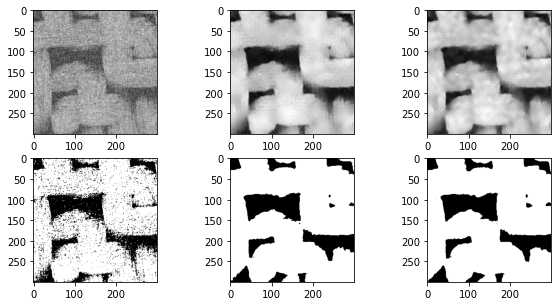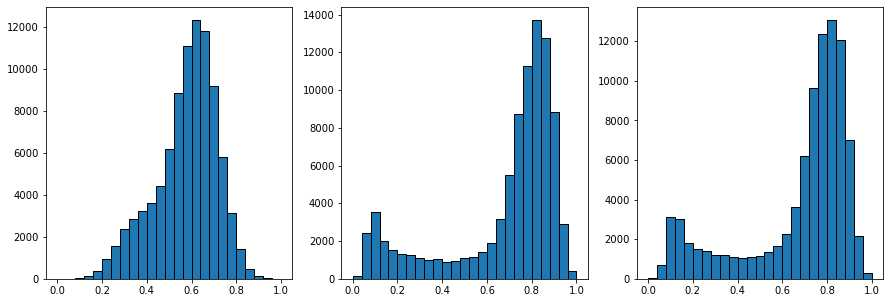### 2.3 孔隙区域分割

Distance Transforms二值图像的距离变换 >() Distance Transforms二值图像的距离变换及其应用_HuiYu-Li的博客-CSDN博客

Distance Transforms Peaks 峰值提取PoreSpy包含一个预定的函数SNOW算法，能够自动应用所有步骤，现在在3D图像上说明各个步骤。

SNOW（subnetwork of the oversegmented watershed）算法基于标记流域的分割算法将图像划分为属于每个孔隙的区域。SNOW 算法的主要贡献是在图像中找到一组合适的初始标记，以便分水岭不会过度分割。

• 1、导入包
import imageio
import numpy as np
import porespy as ps
import openpnm as op
import scipy.ndimage as spim
import matplotlib.pyplot as plt
from porespy.filters import find_peaks, trim_saddle_points, trim_nearby_peaks
from porespy.tools import randomize_colors
from skimage.segmentation import watershed

ps.settings.tqdm['disable'] = True
ps.visualization.set_mpl_style()
np.random.seed(10

class Settings:  # pragma: no cover
r"""
A dataclass for use at the module level to store settings.  This class
is defined as a Singleton so now matter how or where it gets
instantiated the same object is returned, containing all existing
settings.

Parameters
----------
notebook : boolean
Is automatically determined upon initialization of PoreSpy, and is
True if running within a Jupyter notebook and False
otherwise. This is used by the porespy.tools.get_tqdm function
to determine whether a standard or a notebook version of the
progress bar should be used.
tqdm : dict
This dictionary is passed directly to the the tqdm function
throughout PoreSpy (for i in tqdm(range(N), **settings.tqdm)).
To see a list of available options visit the tqdm website.
Probably the most important is 'disable' which when set to
True will silence the progress bars.  It's also possible to
adjust the formatting such as 'colour' and 'ncols', which
controls width.
loglevel : str, or int
Determines what messages to get printed in console. Options are:
"TRACE" (5), "DEBUG" (10), "INFO" (20), "SUCCESS" (25), "WARNING" (30),
"ERROR" (40), "CRITICAL" (50)

"""

def set_mpl_style():  # pragma: no cover
r"""
Prettifies matplotlib's output by adjusting fonts, markersize etc.
"""


2、生成随机的3D纤维图像

#生成200**3，内含200个半径为5的圆柱的3D图像
im = ps.generators.cylinders(shape=[200, 200, 200], r=5, ncylinders=200)
fig, ax = plt.subplots()
ax.imshow(ps.visualization.show_planes(im), cmap=plt.cm.bone);

def cylinders(
shape: List[int],
r: int,
ncylinders: int = None,
porosity: float = None,
phi_max: float = 0,
theta_max: float = 90,
length: float = None,
maxiter: int = 3,
seed=None,
):
r"""
Generates a binary image of overlapping cylinders given porosity OR
number of cylinders.

This is a good approximation of a fibrous mat.

Parameters
----------
shape : list
The size of the image to generate in [Nx, Ny, Nz] where N is the
number of voxels. 2D images are not permitted.
r : scalar
The radius of the cylinders in voxels
ncylinders : int
The number of cylinders to add to the domain. Adjust this value to
control the final porosity, which is not easily specified since
cylinders overlap and intersect different fractions of the domain.
porosity : float
The targeted value for the porosity of the generated mat. The
function uses an algorithm for predicted the number of required
number of cylinder, and refines this over a certain number of
fractional insertions (according to the 'iterations' input).
phi_max : int
A value between 0 and 90 that controls the amount that the
cylinders lie *out of* the XY plane, with 0 meaning all cylinders
lie in the XY plane, and 90 meaning that cylinders are randomly
oriented out of the plane by as much as +/- 90 degrees.
theta_max : int
A value between 0 and 90 that controls the amount of rotation
*in the* XY plane, with 0 meaning all cylinders point in the
X-direction, and 90 meaning they are randomly rotated about the Z
axis by as much as +/- 90 degrees.
length : int
The length of the cylinders to add.  If None (default) then
the cylinders will extend beyond the domain in both directions so
no ends will exist. If a scalar value is given it will be
interpreted as the Euclidean distance between the two ends of the
cylinder. Note that one or both of the ends *may* still lie
outside the domain, depending on the randomly chosen center point
of the cylinder.
maxiter : int
The number of fractional fiber insertions used to target the
requested porosity. By default a value of 3 is used (and this is
typically effective in getting very close to the targeted
porosity), but a greater number can be input to improve the
achieved porosity.
seed : int, optional, default = None
Initializes numpy's random number generator to the specified state. If not
provided, the current global value is used. This means calls to
np.random.state(seed) prior to calling this function will be respected.

Returns
-------
image : ndarray
A boolean array with True values denoting the pore space

Notes
-----
The cylinders_porosity function works by estimating the number of
cylinders needed to be inserted into the domain by estimating
cylinder length, and exploiting the fact that, when inserting any
potentially overlapping objects randomly into a volume v_total (which
has units of pixels and is equal to dimx x dimy x dimz, for example),
such that the total volume of objects added to the volume is v_added
(and includes any volume that was inserted but overlapped with already
occupied space), the resulting porosity will be equal to

After intially estimating the cylinder number and inserting a small
fraction of the estimated number, the true cylinder volume is
calculated, the estimate refined, and a larger fraction of cylinders
inserted. This is repeated a number of times according to the
maxiter argument, yielding an image with a porosity close to
the goal.

Examples
--------
Click here
_
to view online example.

"""


ax.imshow详情参考：3、提取距离变换和峰值

sigma = 0.4
dt = spim.distance_transform_edt(input=im)#提取距离变换
dt1 = spim.gaussian_filter(input=dt, sigma=sigma)#应用高斯模糊过滤噪声
peaks = find_peaks(dt=dt)#提取峰值

print('Initial number of peaks: ', spim.label(peaks))
print('Peaks after trimming saddle points: ', spim.label(peaks))
peaks = trim_nearby_peaks(peaks=peaks, dt=dt)
peaks, N = spim.label(peaks)
print('Peaks after trimming nearby peaks: ', N)
'''
Initial number of peaks:  1539
Peaks after trimming saddle points:  683
Peaks after trimming nearby peaks:  519
'''

def distance_transform_edt(input, sampling=None, return_distances=True,
return_indices=False, distances=None, indices=None):
"""
Exact Euclidean distance transform.

This function calculates the distance transform of the input, by
replacing each foreground (non-zero) element, with its
shortest distance to the background (any zero-valued element).

In addition to the distance transform, the feature transform can
be calculated. In this case the index of the closest background
element to each foreground element is returned in a separate array.

Parameters
----------
input : array_like
Input data to transform. Can be any type but will be converted
into binary: 1 wherever input equates to True, 0 elsewhere.
sampling : float, or sequence of float, optional
Spacing of elements along each dimension. If a sequence, must be of
length equal to the input rank; if a single number, this is used for
all axes. If not specified, a grid spacing of unity is implied.
return_distances : bool, optional
Whether to calculate the distance transform.
Default is True.
return_indices : bool, optional
Whether to calculate the feature transform.
Default is False.
distances : float64 ndarray, optional
An output array to store the calculated distance transform, instead of
returning it.
return_distances must be True.
It must be the same shape as input.
indices : int32 ndarray, optional
An output array to store the calculated feature transform, instead of
returning it.
return_indicies must be True.
Its shape must be (input.ndim,) + input.shape.

Returns
-------
distances : float64 ndarray, optional
The calculated distance transform. Returned only when
return_distances is True and distances is not supplied.
It will have the same shape as the input array.
indices : int32 ndarray, optional
The calculated feature transform. It has an input-shaped array for each
dimension of the input. See example below.
Returned only when return_indices is True and indices is not
supplied.

Notes
-----
The Euclidean distance transform gives values of the Euclidean
distance::

n
y_i = sqrt(sum (x[i]-b[i])**2)
i

where b[i] is the background point (value 0) with the smallest
Euclidean distance to input points x[i], and n is the
number of dimensions.

Examples
--------
>>> from scipy import ndimage
>>> import numpy as np
>>> a = np.array(([0,1,1,1,1],
...               [0,0,1,1,1],
...               [0,1,1,1,1],
...               [0,1,1,1,0],
...               [0,1,1,0,0]))
>>> ndimage.distance_transform_edt(a)
array([[ 0.    ,  1.    ,  1.4142,  2.2361,  3.    ],
[ 0.    ,  0.    ,  1.    ,  2.    ,  2.    ],
[ 0.    ,  1.    ,  1.4142,  1.4142,  1.    ],
[ 0.    ,  1.    ,  1.4142,  1.    ,  0.    ],
[ 0.    ,  1.    ,  1.    ,  0.    ,  0.    ]])

With a sampling of 2 units along x, 1 along y:

>>> ndimage.distance_transform_edt(a, sampling=[2,1])
array([[ 0.    ,  1.    ,  2.    ,  2.8284,  3.6056],
[ 0.    ,  0.    ,  1.    ,  2.    ,  3.    ],
[ 0.    ,  1.    ,  2.    ,  2.2361,  2.    ],
[ 0.    ,  1.    ,  2.    ,  1.    ,  0.    ],
[ 0.    ,  1.    ,  1.    ,  0.    ,  0.    ]])

>>> edt, inds = ndimage.distance_transform_edt(a, return_indices=True)
>>> inds
array([[[0, 0, 1, 1, 3],
[1, 1, 1, 1, 3],
[2, 2, 1, 3, 3],
[3, 3, 4, 4, 3],
[4, 4, 4, 4, 4]],
[[0, 0, 1, 1, 4],
[0, 1, 1, 1, 4],
[0, 0, 1, 4, 4],
[0, 0, 3, 3, 4],
[0, 0, 3, 3, 4]]])

With arrays provided for inplace outputs:

>>> indices = np.zeros(((np.ndim(a),) + a.shape), dtype=np.int32)
>>> ndimage.distance_transform_edt(a, return_indices=True, indices=indices)
array([[ 0.    ,  1.    ,  1.4142,  2.2361,  3.    ],
[ 0.    ,  0.    ,  1.    ,  2.    ,  2.    ],
[ 0.    ,  1.    ,  1.4142,  1.4142,  1.    ],
[ 0.    ,  1.    ,  1.4142,  1.    ,  0.    ],
[ 0.    ,  1.    ,  1.    ,  0.    ,  0.    ]])
>>> indices
array([[[0, 0, 1, 1, 3],
[1, 1, 1, 1, 3],
[2, 2, 1, 3, 3],
[3, 3, 4, 4, 3],
[4, 4, 4, 4, 4]],
[[0, 0, 1, 1, 4],
[0, 1, 1, 1, 4],
[0, 0, 1, 4, 4],
[0, 0, 3, 3, 4],
[0, 0, 3, 3, 4]]])

"""

#trim_saddle_points()
r"""
Removes peaks that were mistakenly identified because they lied on a
saddle or ridge in the distance transform that was not actually a true
local peak.

Parameters
----------
peaks : ndarray
A boolean image containing True values to mark peaks in the
distance transform (dt)
dt : ndarray
The distance transform of the pore space for which the peaks
are sought.
maxiter : int
The number of iteration to use when finding saddle points.
The default value is 20.

Returns
-------
image : ndarray
An image with fewer peaks than the input image

References
----------
 Gostick, J. "A versatile and efficient network extraction algorithm
using marker-based watershed segmentation".  Physical Review E. (2017)

Examples
--------
Click here
_
to view online example.

"""

#trim_nearby_peaks()
def trim_nearby_peaks(peaks, dt, f=1):
r"""
Removes peaks that are nearer to another peak than to solid

Parameters
----------
peaks : ndarray
A image containing nonzeros values indicating peaks in the distance
transform (dt).  If peaks is boolean, a boolean is returned;
if peaks have already been labelled, then the original labels
are returned, missing the trimmed peaks.
dt : ndarray
The distance transform of the pore space
f : scalar
Controls how close peaks must be before they are considered near
to each other. Sets of peaks are tagged as too near if
d_neighbor < f * d_solid.

Returns
-------
image : ndarray
An array the same size and type as peaks containing a subset of
the peaks in the original image.

Notes
-----
Each pair of peaks is considered simultaneously, so for a triplet of nearby
peaks, each pair is considered.  This ensures that only the single peak
that is furthest from the solid is kept.  No iteration is required.

References
----------
 Gostick, J. "A versatile and efficient network extraction
algorithm using marker-based watershed segmenation". Physical Review
E. (2017)

Examples
--------
Click here
_
to view online example.

"""


regions = watershed(image=-dt, markers=peaks, mask=dt > 0)
regions = randomize_colors(regions)#赋予不同孔隙区域不同的颜色
fig, ax = plt.subplots()
ax.imshow((regions*im)[:, :, 100], cmap=plt.cm.nipy_spectral);

#watershed()
def watershed(image, markers=None, connectivity=1, offset=None, mask=None,
compactness=0, watershed_line=False):
"""Find watershed basins in image flooded from given markers.

Parameters
----------
image : ndarray (2-D, 3-D, ...)
Data array where the lowest value points are labeled first.
markers : int, or ndarray of int, same shape as image, optional
The desired number of markers, or an array marking the basins with the
values to be assigned in the label matrix. Zero means not a marker. If
None (no markers given), the local minima of the image are used as
markers.
connectivity : ndarray, optional
An array with the same number of dimensions as image whose
non-zero elements indicate neighbors for connection.
Following the scipy convention, default is a one-connected array of
the dimension of the image.
offset : array_like of shape image.ndim, optional
offset of the connectivity (one offset per dimension)
mask : ndarray of bools or 0s and 1s, optional
Array of same shape as image. Only points at which mask == True
will be labeled.
compactness : float, optional
Use compact watershed _ with given compactness parameter.
Higher values result in more regularly-shaped watershed basins.
watershed_line : bool, optional
If watershed_line is True, a one-pixel wide line separates the regions
obtained by the watershed algorithm. The line has the label 0.

Returns
-------
out : ndarray
A labeled matrix of the same type and shape as markers

--------
skimage.segmentation.random_walker : random walker segmentation
A segmentation algorithm based on anisotropic diffusion, usually
slower than the watershed but with good results on noisy data and
boundaries with holes.

Notes
-----
This function implements a watershed algorithm _ _ that apportions
pixels into marked basins. The algorithm uses a priority queue to hold
the pixels with the metric for the priority queue being pixel value, then
the time of entry into the queue - this settles ties in favor of the
closest marker.

Some ideas taken from
Soille, "Automated Basin Delineation from Digital Elevation Models Using
Mathematical Morphology", Signal Processing 20 (1990) 171-182

The most important insight in the paper is that entry time onto the queue
solves two problems: a pixel should be assigned to the neighbor with the
largest gradient or, if there is no gradient, pixels on a plateau should
be split between markers on opposite sides.

This implementation converts all arguments to specific, lowest common
denominator types, then passes these to a C algorithm.

Markers can be determined manually, or automatically using for example
the local minima of the gradient of the image, or the local maxima of the
distance function to the background for separating overlapping objects
(see example).

References
----------
..  https://en.wikipedia.org/wiki/Watershed_%28image_processing%29

..  http://cmm.ensmp.fr/~beucher/wtshed.html

..  Peer Neubert & Peter Protzel (2014). Compact Watershed and
Preemptive SLIC: On Improving Trade-offs of Superpixel Segmentation
Algorithms. ICPR 2014, pp 996-1001. :DOI:10.1109/ICPR.2014.181
https://www.tu-chemnitz.de/etit/proaut/publications/cws_pSLIC_ICPR.pdf

Examples
--------
The watershed algorithm is useful to separate overlapping objects.

We first generate an initial image with two overlapping circles:

>>> x, y = np.indices((80, 80))
>>> x1, y1, x2, y2 = 28, 28, 44, 52
>>> r1, r2 = 16, 20
>>> mask_circle1 = (x - x1)**2 + (y - y1)**2 >> mask_circle2 = (x - x2)**2 + (y - y2)**2 >> image = np.logical_or(mask_circle1, mask_circle2)

Next, we want to separate the two circles. We generate markers at the
maxima of the distance to the background:

>>> from scipy import ndimage as ndi
>>> distance = ndi.distance_transform_edt(image)
>>> from skimage.feature import peak_local_max
>>> max_coords = peak_local_max(distance, labels=image,
...                             footprint=np.ones((3, 3)))
>>> local_maxima = np.zeros_like(image, dtype=bool)
>>> local_maxima[tuple(max_coords.T)] = True
>>> markers = ndi.label(local_maxima)

Finally, we run the watershed on the image and markers:

>>> labels = watershed(-distance, markers, mask=image)

The algorithm works also for 3-D images, and can be used for example to
separate overlapping spheres.
"""net = ps.networks.regions_to_network(regions*im, voxel_size=1)
pn = op.io.network_from_porespy(net)
print(pn)
ax = op.visualization.plot_coordinates(pn)
ax = op.visualization.plot_connections(pn, ax=ax)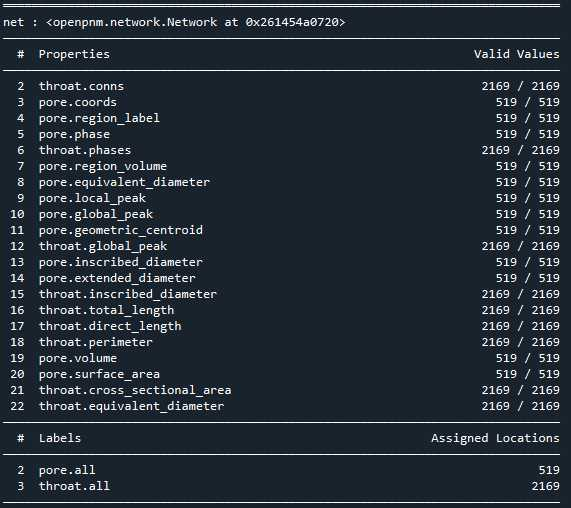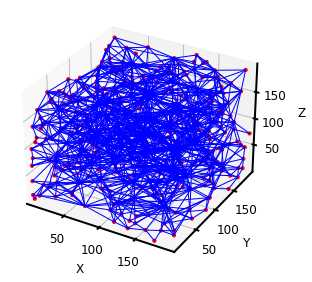PoreSpy提取的孔隙网络可以直接导入OpenPNM进行渗透率计算，但是通过对比可以发现两者网络模型的相关参数有些许差异，而且与常用的最大球提取法不同，PoreSpy采用SNOW算法提取网络模型，而PoreSpy内置函数里只能计算菲克扩散的扩散系数，没有计算渗透系数的功能，所以具体能否用PoreSpy导出的网络计算渗透率还存疑